Creating an organic grid on a sphere

Interactive grid on a sphere made in the Godot Engine. Left-click to place blocks and right-click to remove them. Click and drag to rotate the sphere.

I recently created a tweet about creating an organic looking quad grid on a sphere and it got rather popular.

Since I got some feedback and people asking me how to do this, I decided to give some more context. However, don’t expect a full-on tutorial for the engine of your choice. Instead, this is supposed to give you some more background on how to implement something like this and explain some of the theory behind it. I will attempt to cover the grid generation itself as well as give a brief introduction to tile placement and marching squares.

As is probably already clear to you, this is directly based on a grid generation algorithm that Oskar Stålberg posted on Twitter a while ago and which he uses in his sandbox town building game Townscaper. He has also written a very in-depth post on the process of building the tech behind Townscaper on imgur.

This was, however, only presented for a grid in a plane so far. What do we need to do to adapt it for a sphere (or even crazier shapes)?

The surprisingly simple answer is: nothing.

Grid Generation

To summarize the algorithm:

1. Start with a triangulation (in the tweeet above triangles in a hexagon)
2. Remove random edges triangles to create quads (we will now have a grid consisting of mostly quads but also some triangles)
4. Relax the grid to make it look more natural

Step 1: Triangulation

The first step of the algorithm requires a triangulation. What is a triangulation, you ask? Well, there are many definitions (including but not limited to tracking bad guys in police crime dramas) but in our case we will define it as surface triangulation which, with the words of Wikipedia, is “a net of triangles, which covers a given surface partly or totally”. Simply put, we split our initial shape into many smaller triangles. In the tweet above this is the triangulation of a hexagon but we can triangulate any surface, really.

A popular triangulation of the surface of a sphere is the so-called icosphere. It is, of course, not the only triangulation but it has some nice properties including the fact that all triangles have the same size.

Which Software Should You Use?

I am doing all the grid generation in Blender, mainly because Blender’s bmesh module is a breeze to work with and already has most operations (like removing edges, subdividing faces, etc.) implemented. Of course, you can do all this using whichever software or tool you prefer (and even directly in-engine if you want to do the grid generation in real-time).

Below is an image of our icosphere in Blender: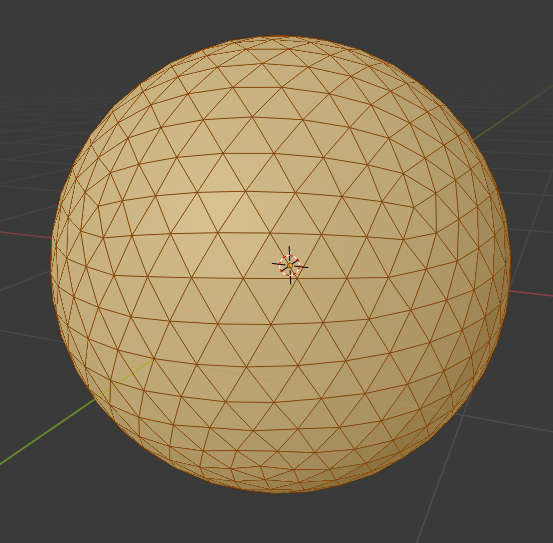Our starting triangulation, the icosphere.

Step 2: Removing Edges

Removing edges is relatively straightforward. However, we need to be careful to keep track of which edges we have already removed as to not accidentally remove an edge of a face that is already a quad. The rule for this is simple: when we remove an edge between to faces, we can’t remove any of the remaining six edges of the two now merged faces anymore. I solved this by keeping track of a list of edges that are stil “available” and only removing edges listed there from the mesh. When I remove an edge from the mesh, I remove the now forbidden six edges from the list.

If we did everything right, our former icosphere should look something like this:The mesh after the edge removal step.

Step 3: Subdivide Faces

We are currently facing one problem: Our result should be a quad mesh. That is, a mesh consisting only of faces with four edges. But there are still some triangles left in our current mesh. Luckily, triangles can easily subdivided into three smaller quads as illustrated in figure 3. In the same way, the already existing quads can be subdivided into four smaller quads as well. By doing this, we thus end up with a mesh consisting only of quads.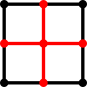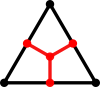Done correctly, our mesh now looks like this: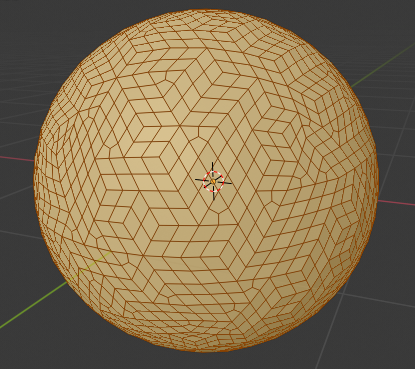The mesh with all faces subdivided into quads.

Step 4: Mesh Relaxation

We are now almost where we want to be but the mesh does not look very organic yet. This is where the mesh relaxation comes in. For me, this was the trickiest step since it involved some experimentation to get it to look just right.

In the simpler case of a grid in a plane, there are some ressources available which outline possible relaxation methods, like this article from andersource.dev, but most of them seem to involve letting each quad force its vertices into a square shape.

I see no reason why an approach like this shouldn’t work on a spherical mesh as well. However, I approached the problem from a slightly different angle and started with (a restricted version of) Laplacian smoothing which is generally used to smooth meshes. In the end I needed to augment this by adding forces which try to prevent too small/large and thin quads as well. There are probably many good ways too relax the mesh but some good guidelines should be:

• each quad should be as square as possible
• all quads should have approximately the same area
• or any similar criteria

Using my approach, the final result looks like this: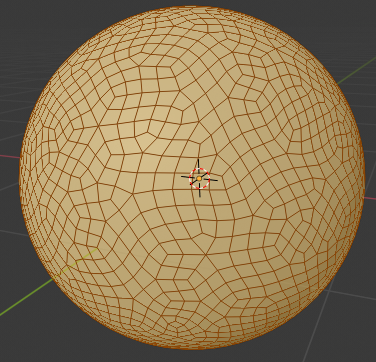✨ The final mesh! ✨

Bonus: Tiles and Marching Squares

Deforming the Tiles

So now we have our mesh. But what can we actually do with it? I decided to go for a very minimalistic Townscaper “clone” which uses the sphere mesh for tile placement. Call it SphereScaper or an equally creative name.

One advantage of a quad mesh is that we can place deformed square tiles at each face. The math for this is relatively simple. We only need to deform from our mesh’s $$x$$- and $$y$$-coordinates (assuming $$z$$ is up) since we can just extrude the $$z$$ component along the face’s normal vector.

Now let’s assume that our tile’s corner points are $$\vec{a} = (0, 0)$$, $$\vec{b} = (1, 0)$$, $$\vec{c} = (0, 1)$$ and $$\vec{d} = (1, 1)$$ (that is, our tile is defined inside a unit square). An arbitrary quad face on our mesh is defined by four corner vertices in 3D space, let’s call them $$\vec{a}'$$, $$\vec{b}'$$, $$\vec{c}'$$ and $$\vec{d}'$$.

Each coordinate $$\vec{p} = (x, y)$$ in the original tile can be described by the bilinear parametrisation

\begin{align} \vec{p} &= (1 - x) \cdot (1 - y) \cdot \vec{a} + x \cdot (1 - y) \cdot \vec{b} + (1 - x) \cdot y \cdot \vec{c} + x \cdot y \cdot \vec{d} \\ &= \vec{a} + x \cdot (1 - y) \cdot (\vec{b} - \vec{a}) + (1 - x) \cdot y \cdot (\vec{c} - \vec{a}) + x \cdot y \cdot (\vec{d} - \vec{a}) \end{align}

We can easily transform this to the new quad:

\begin{align} \vec{p}' &= \vec{a}' + x \cdot (1 - y) \cdot (\vec{b}' - \vec{a}') + (1 - x) \cdot y \cdot (\vec{c}' - \vec{a}') + x \cdot y \cdot (\vec{d}' - \vec{a}') \end{align}

where $$\vec{p}'$$ is the resulting coordinate inside the quad.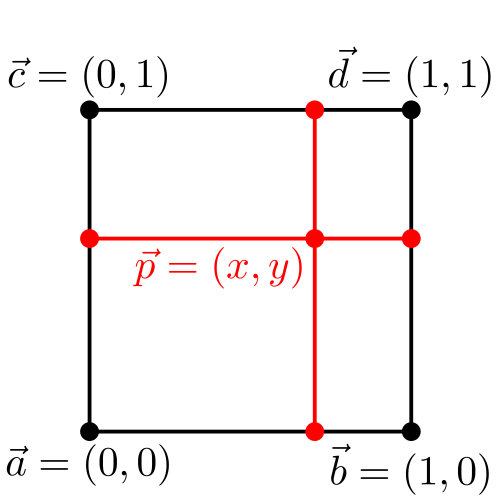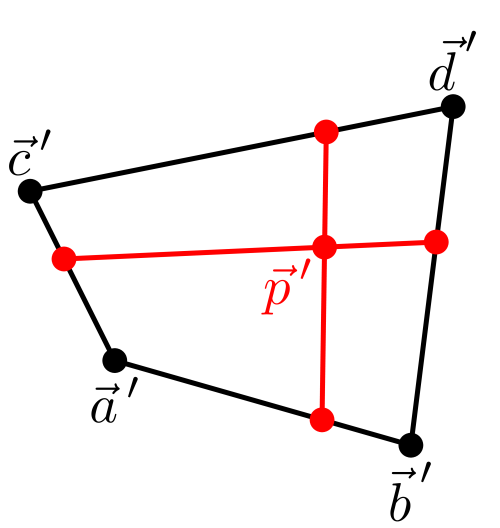Transforming the point $$\vec{p}$$ to the point $$\vec{p}'$$ inside the new quad.

We can also easily rotate tiles by reordering the new quads’ corners.

Coordinate Systems

Pay attention to the coordinate system your tool or engine is using. The Godot engine, for example, uses a different coordinate convention than Blender so the order and sign of the vertex coordinates of my tile meshes change on import to Godot. In this case, the code I use to deform the tile looks like this ($$y$$ and $$z$$ are switched and $$z$$ changes sign as well):

var mdt = MeshDataTool.new()
...
var v = mdt.get_vertex(i)
...
v = a + v.x * (1.0 + v.z) * (b - a) - (1.0 - v.x) * v.z * (c - a) - v.x * v.z * (d - a) + v.normalized() * v.y
...

Since the grid is spherical I can also extrude along the normalized vertex position vector v.normalized() which is more accurate than using face normals.

Below is a nice figure illustrating the coordinate conventions used by different engines and softwares:Coordinate system conventions used by different softwares. Source: @FreyaHolmer.

A Brief Introduction to Marching Squares

The most straightforward way of placing tiles on a grid or mesh is to assign values to each face of said grid and then draw the corresponding tiles. For very simple cases, this may work. However, you will quickly run into problems when you want to draw transitions between tiles, for example a shoreline between water and land. A very popular way of solving this is to use marching squares.

In marching squares, values are not assigned to the individual faces but to the mesh vertices instead. For each quad face we then read the values of its four corner vertices and choose the corresponding tile. In case of a simple configuration with two values like “land” and “water” we need to check for a total of 16 cases per cell. Since many of these are just rotated versions of other cases we only need to create a total of 6 different tiles to make marching squares run. (There are other algorithms which use more diverse tilesets as well like the blob tileset but most of them boil down to the same concept.)Look-up table with all possible face configurations and their corresponding tile drawn in. Solid vertices could e.g. correspond to land while empty vertices are water.

Despite its name marchings squares works without modifications on any quadrilateral mesh so there is no downside to using it on an irregular grid. It even allows building non-rectangular structures with ease since the vertices do not neccessarily need to have exactly four neighbours. In my oppinion, this improves the organic feeling a lot.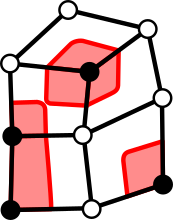Example for applying the marching squares algorithm to an irregular grid using the look-up table from above.

I don’t want to delve much deeper here but in case you want to know more about marching squares, I found the Wikipedia article to be surprisingly helpful and there are lots of tutorials explicitly on the topic out there.Example tile modeled in Blender.

Marching Cubes

You may or may not have heard of marching cubes, marching square’s big brother. It translates the same concept to three dimensions (with 256 possible configurations per cube with 15 unique tiles required). You can use it to allow the player to built upwards and create towers and much more.